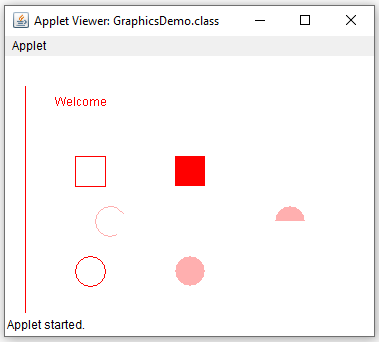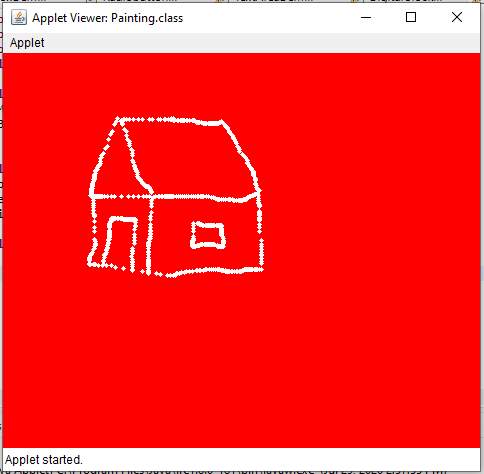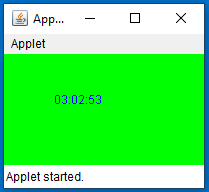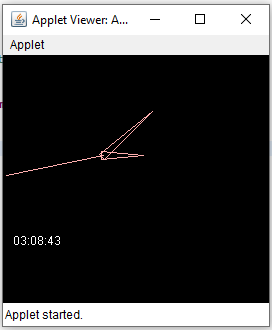# Graphics in Applet

## Graphics in Applet with Examples

In this article, I am going to discuss Graphics in Apple with Examples. Please read our previous article, where we discussed Applet in Java. At the end of this article, you will understand what are graphics and when and how to use Graphics in Applet with examples.

##### Graphics in Applet:

All graphics are drawn relative to a window. This can be the main window of an applet, a child window of an applet, or a stand-alone application window. The origin of each window is at the top-left Coordinates 0,0. Coordinates are specified in pixels. All output to a window takes place through a graphics context. A graphics context is encapsulated by the Graphics class and is obtained in two ways:

1. It is passed to an applet when one of its various methods, such as paint() or update(), is called.
2. It is returned by the getGraphics( ) method of Component.

The Graphics class defines a number of drawing functions. Each shape can be drawn edge-only or filled. Objects are drawn and filled in the currently selected graphics color, which is black by default. When a graphics object is drawn that exceeds the dimensions of the window, the output is automatically clipped.

java.awt.Graphics class provides many methods for graphics programming.

##### Methods of Graphics class
1. public abstract void drawString(String str, int x, int y): is used to draw the specified string.
2. public void drawRect(int x, int y, int width, int height): draw a rectangle with the specified width and height.
3. public abstract void fillRect(int x, int y, int width, int height): is used to fill the rectangle with the default color and specified width and height.
4. public abstract void drawOval(int x, int y, int width, int height): is used to draw an oval with the specified width and height.
5. public abstract void fillOval(int x, int y, int width, int height): is used to fill an oval with the default color and specified width and height.
6. public abstract void drawLine(int x1, int y1, int x2, int y2): is used to draw a line between the points(x1, y1) and (x2, y2).
7. public abstract boolean drawImage(Image img, int x, int y, ImageObserver observer): is used to draw the specified image.
8. public abstract void drawArc(int x, int y, int width, int height, int startAngle, int arcAngle): is used to draw a circular or elliptical arc.
9. public abstract void fillArc(int x, int y, int width, int height, int startAngle, int arcAngle): is used to fill a circular or elliptical arc.
10. public abstract void setColor(Color c): is used to set the graphics current color to the specified color.
11. public abstract void setFont(Font font): is used to set the graphics current font to the specified font.
##### Examples to Understand Graphics in Applet:
```import java.applet.Applet;
import java.awt.*;

/*
<applet code="GraphicsDemo.class" width="300" height="300">
</applet>
*/
public class GraphicsDemo extends Applet
{
public void paint (Graphics g)
{
g.setColor (Color.red);
g.drawString ("Welcome", 50, 50);
g.drawLine (20, 30, 20, 300);
g.drawRect (70, 100, 30, 30);
g.fillRect (170, 100, 30, 30);
g.drawOval (70, 200, 30, 30);

g.setColor (Color.pink);
g.fillOval (170, 200, 30, 30);
g.drawArc (90, 150, 30, 30, 30, 270);
g.fillArc (270, 150, 30, 30, 0, 180);
}
}```
###### Output:##### Example: Painting in Applet
```import java.awt.*;
import java.awt.event.*;
import java.applet.*;
public class Painting extends Applet implements MouseMotionListener
{
public void init ()
{
setBackground (Color.red);
}

public void mouseDragged (MouseEvent me)
{
Graphics g = getGraphics ();
g.setColor (Color.white);
g.fillOval (me.getX (), me.getY (), 5, 5);
}
public void mouseMoved (MouseEvent me)
{
}
}```
###### Output:##### Example to Display Digital Clock in an Applet
```import java.applet.*;
import java.awt.*;
import java.util.*;
import java.text.*;
/*
<applet code="DigitalClock" width="300" height="300">
</applet>
*/
public class DigitalClock extends Applet implements Runnable
{
int hours = 0, minutes = 0, seconds = 0;
String timeString = "";

public void init ()
{
setBackground (Color.green);
}

public void start ()
{
t.start ();
}

public void run ()
{
try
{
while (true)
{
Calendar cal = Calendar.getInstance ();
hours = cal.get (Calendar.HOUR_OF_DAY);
if (hours > 12)
hours -= 12;
minutes = cal.get (Calendar.MINUTE);
seconds = cal.get (Calendar.SECOND);

SimpleDateFormat formatter = new SimpleDateFormat ("hh:mm:ss");
Date date = cal.getTime ();
timeString = formatter.format (date);

repaint ();
t.sleep (1000);	// interval given in milliseconds
}
}
catch (Exception e)
{
}
}

public void paint (Graphics g)
{
g.setColor (Color.blue);
g.drawString (timeString, 50, 50);
}
}```
###### Output:##### Example to Display Analog Clock in Applet
```import java.applet.*;
import java.awt.*;
import java.util.*;
import java.text.*;
/*
<applet code="AnalogClock" width="300" height="300">
</applet>
*/
public class AnalogClock extends Applet implements Runnable
{
int width, height;
int hours = 0, minutes = 0, seconds = 0;
String timeString = "";
public void init ()
{
width = getSize ().width;
height = getSize ().height;
setBackground (Color.black);
}
public void start ()
{
if (t == null)
{
t.start ();
}
else
{
{
synchronized (this)
{
notify ();
}
}
}
}

public void stop ()
{
}

public void run ()
{
try
{
while (true)
{
Calendar cal = Calendar.getInstance ();
hours = cal.get (Calendar.HOUR_OF_DAY);
if (hours > 12)
hours -= 12;
minutes = cal.get (Calendar.MINUTE);
seconds = cal.get (Calendar.SECOND);
SimpleDateFormat formatter =
new SimpleDateFormat ("hh:mm:ss", Locale.getDefault ());
Date date = cal.getTime ();
timeString = formatter.format (date);
{
synchronized (this)
{
{
wait ();
}
}
}
repaint ();
t.sleep (1000);
}
}
catch (Exception e)
{
}
}

void drawHand (double angle, int radius, Graphics g)
{
angle -= 0.5 * Math.PI;
int x = (int) (radius * Math.cos (angle));
int y = (int) (radius * Math.sin (angle));
g.drawLine (width / 2, height / 2, width / 2 + x, height / 2 + y);
}

void drawWedge (double angle, int radius, Graphics g)
{
angle -= 0.5 * Math.PI;
int x = (int) (radius * Math.cos (angle));
int y = (int) (radius * Math.sin (angle));
angle += 2 * Math.PI / 3;
int x2 = (int) (5 * Math.cos (angle));
int y2 = (int) (5 * Math.sin (angle));
angle += 2 * Math.PI / 3;
int x3 = (int) (5 * Math.cos (angle));
int y3 = (int) (5 * Math.sin (angle));
g.drawLine (width / 2 + x2, height / 2 + y2, width / 2 + x, height / 2 + y);
g.drawLine (width / 2 + x3, height / 2 + y3, width / 2 + x, height / 2 + y);
g.drawLine (width / 2 + x2, height / 2 + y2, width / 2 + x3, height / 2 + y3);
}

public void paint (Graphics g)
{
g.setColor (Color.pink);
drawWedge (2 * Math.PI * hours / 12, width / 5, g);
drawWedge (2 * Math.PI * minutes / 60, width / 3, g);
drawHand (2 * Math.PI * seconds / 60, width / 2, g);
g.setColor (Color.white);
g.drawString (timeString, 10, height - 10);
}
}```
###### Output:In the next article, I am going to discuss AWT in Java with examples. Here, in this article, I try to explain Graphics in Applet with Examples and I hope you enjoy this Graphics in Applet with Examples article.